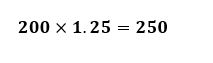# How to add percentages together

Want to find out how to add percentages together? We've provided a step-by-step guide of how to add percentages together to teach you everything you need to know.## Step 1: Add 100% and the given percentage

So if we want to add 15% of 240, we first add 100 and 15.

## Step 2: Change the percentage to a decimal

Change the percentage to a decimal by moving two decimal places to the left of the Number (base value).

## Step 3: Multiply the decimal by the base value

Since the percentage is already changed to its decimal form, multiply the decimal by the base value.

That’s why if we add 15% of 240 to itself, the result would be 276.

## Examples on adding percentages together

Q1) Chuck wants to increase his monthly savings by 25%. If he used to save \$200 every month, how much he be saving for the next months?

To add 25% to 200, we first add 100 and 15: 100+15=115

Change the percentage to its decimal form by moving two decimal places to left of the percentage.Once we have the decimal form of the percentage, multiply it to the given base value.Hence, Chuck would need to save \$250 the next months.

#### Join today

The fastest way to practice

Unlock our complete testing platform and improve faster that ever.